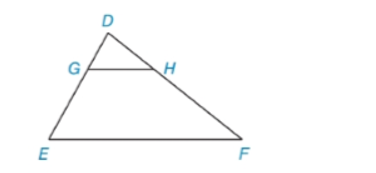Chapter 5.3, Problem 12EElementary Geometry For College St...

7th Edition
Alexander + 2 others
ISBN: 9781337614085

Solutions

Chapter
SectionElementary Geometry For College St...

7th Edition
Alexander + 2 others
ISBN: 9781337614085
Textbook Problem

In Exercises 9 to 12, name the method that explains why ∆ D G H ~ ∆ D E F . ∠ D G H ≅ ∠ D E FTo determine

To name:

The method that explains why DGH~DEF

Explanation

Given:

The triangle,

Here,

DGHDEF

Approach:

Two polygons are similar if and only if two conditions are satisfied.

1. All pairs of corresponding angles are congruent.

2. All pairs of corresponding sides are proportional.

Definitions:

AA:

If the two angles of one triangle are congruent to the two angles of a another triangle, then the triangles are similar.

SAS:

If an angle of one triangle is congruent to an angle of a second triangle and the pairs of sides including the angles are proportional (in length), then the triangles are similar.

SSS:

If the three sides of one triangle are proportional (in length) to the three corresponding sides of a second triangle, then the triangles are similar.

Description:

For the given triangle,

It is given that,

DGHDEF

The given figure contains two triangles DGH and DEF

Still sussing out bartleby?

Check out a sample textbook solution.

See a sample solution

The Solution to Your Study Problems

Bartleby provides explanations to thousands of textbook problems written by our experts, many with advanced degrees!

Get Started

Sketch the graph of y=2x1.

Calculus: An Applied Approach (MindTap Course List)

What is the defining characteristic of a repeated-measures or within-subjects research design?

Essentials of Statistics for The Behavioral Sciences (MindTap Course List)

In Exercises 4562, find the values of x that satisfy the inequality (inequalities). 54. x 4 1 and x + 3 2

Applied Calculus for the Managerial, Life, and Social Sciences: A Brief Approach

If limx0sinxx=1, the angle x must be measured in: a) radians b) degrees c) it does not matter

Study Guide for Stewart's Single Variable Calculus: Early Transcendentals, 8th

True or False: , for –π ≤ t ≤ π, is smooth.

Study Guide for Stewart's Multivariable Calculus, 8th